# SN Dey Class 11 Solutions Limits Very Short Answer Type Questions

SN Dey Class 11 Solutions | SN Dey Class 11 Limits Solutions | Solution of SN Dey Class 11 Limits Very Short Answer Type Questions | West Bengal Board Class 11 Limit Solution | SN Dey Class 11 Math Chapter Limit Solution | Limits Very Short Answer Type Questions Answers

## SN Dey Class 11 Limits Very Short Answer Type Questions Solutions

SN Dey class 11 Limits very short answer type questions Ex 1 solutions:

Ex 1: Give the definition of limit of a function.

Solution: Let $f(x)$ be a function of $x$. We say $\lim\limits_{x \to a}f(x)=L$ if the following is satisfied.

$\lim\limits_{x \to a-}f(x)=\lim\limits_{x \to a+}f(x)=L$.

In other words, both the left-hand limit and right-hand limit of $f(x)$ as $x$ tends to $a$ is equal to $L$, then we have$\lim\limits_{x \to a}f(x)=L$.

SN Dey class 11 Limits very short answer type questions Ex 2 solutions:

Ex 2: Distinguish between $\lim\limits_{x \to a} f(x)$ and $f(a)$.

Solution:

(i) $\lim\limits_{x \to a} f(x)$ denotes the limiting value of $f(x)$ when $x$ tends to $a$, whereas $f(a)$ is the functional value of $f(x)$ at the point $x=a$.

(ii) It may happen that $\lim\limits_{x \to a} f(x) \neq f(a)$, but if the function $f(x)$ is continous at $x=a$, then they must be equal.

SN Dey class 11 Limits very short answer type questions 3 solutions: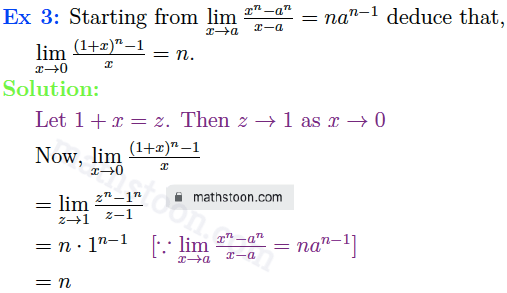SN Dey class 11 Limits very short answer type questions 5 and 6 solutions: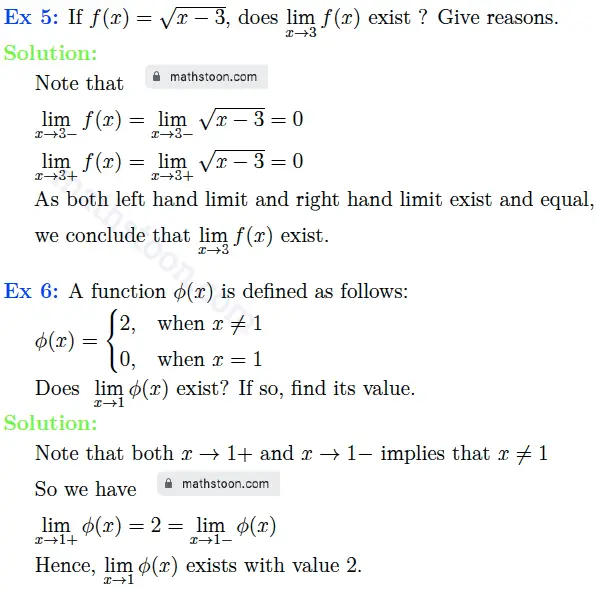SN Dey class 11 Limits very short answer type questions 7 solutions: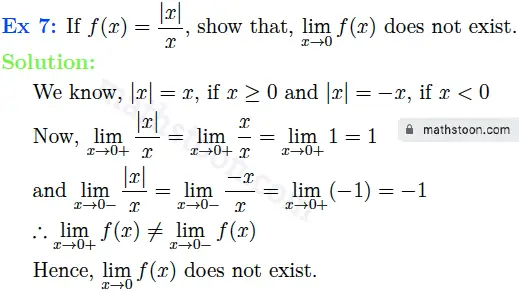SN Dey class 11 Limits very short answer type questions 8.(i), (ii) solutions: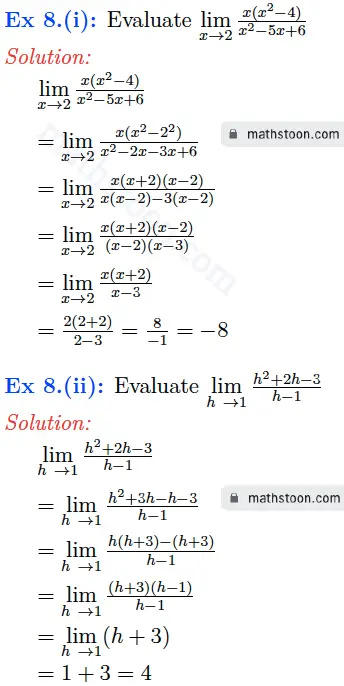SN Dey class 11 Limits very short answer type questions 8.(iii) solutions: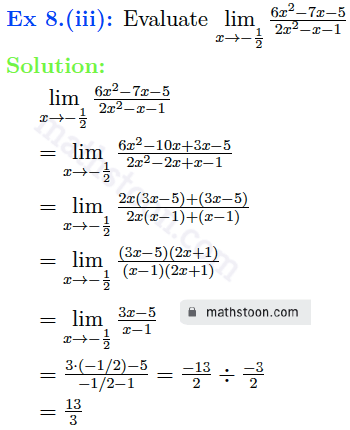SN Dey class 11 Limits very short answer type questions 8.(iv) solutions: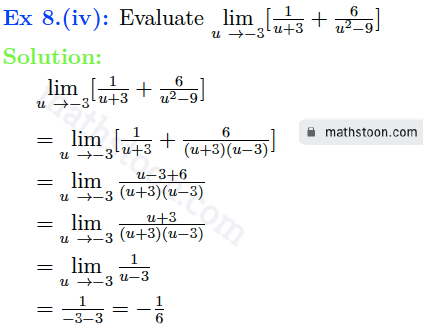SN Dey class 11 Limits very short answer type questions 8.(v), (vi) solutions: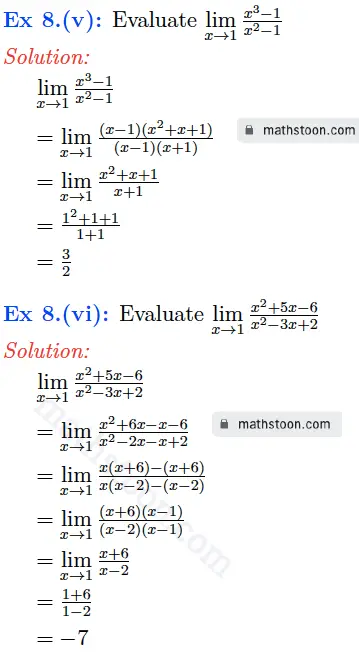SN Dey class 11 Limits very short answer type questions 8.(vii) solutions: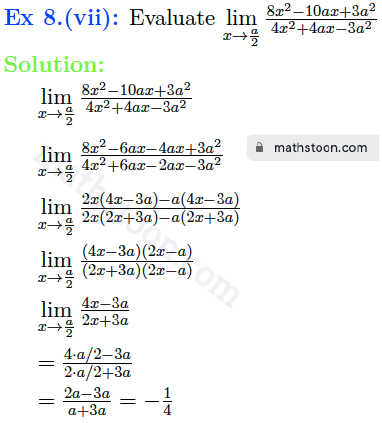SN Dey class 11 Limits very short answer type questions 8.(viii) solutions: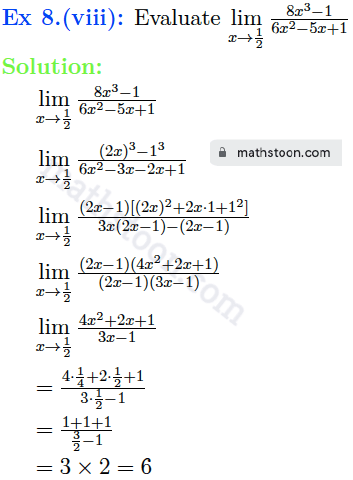SN Dey class 11 Limits very short answer type questions 9.(i) solutions: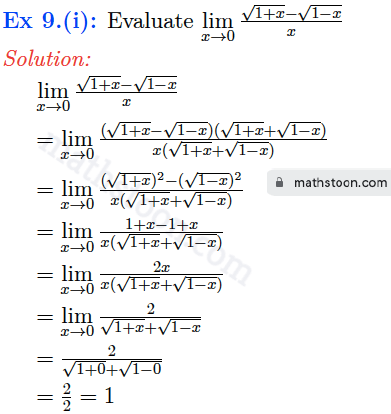SN Dey class 11 Limits very short answer type questions 9.(ii) solutions: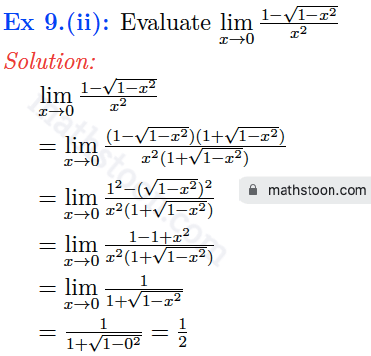SN Dey class 11 Limits very short answer type questions 9.(iii) solutions: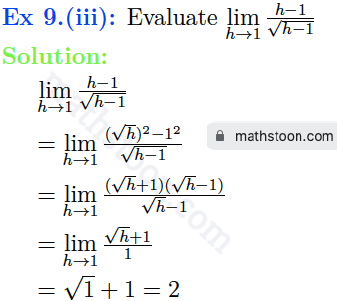SN Dey class 11 Limits very short answer type questions 9.(iv) solutions: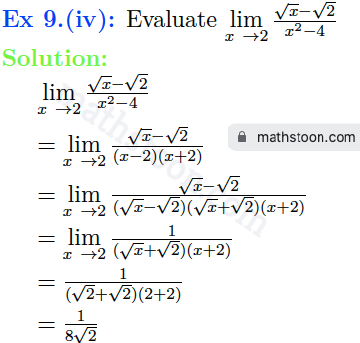SN Dey class 11 Limits very short answer type questions 9.(v) solutions: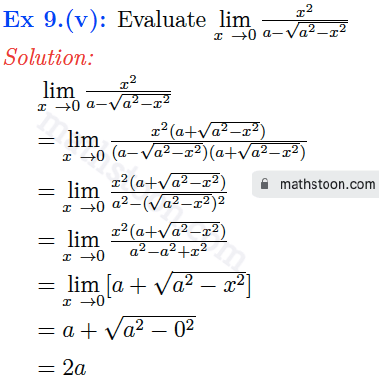SN Dey class 11 Limits very short answer type questions 9.(vi) solutions: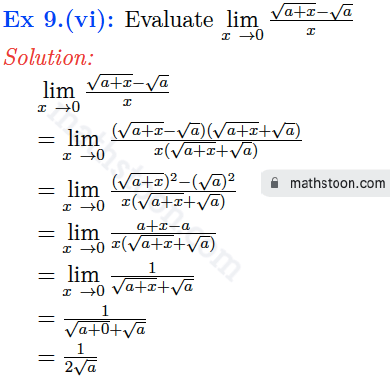SN Dey class 11 Limits very short answer type questions 9.(vii) solutions: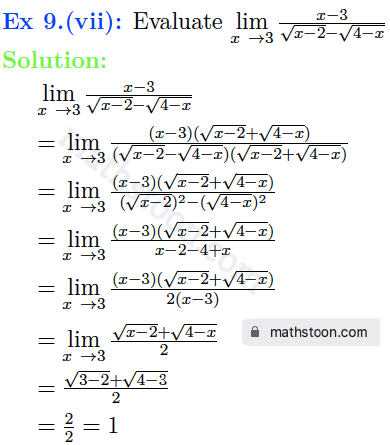SN Dey class 11 Limits very short answer type questions 9.(viii) solutions: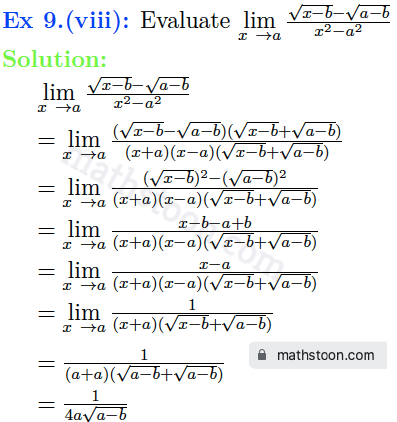SN Dey class 11 Limits very short answer type questions 9.(ix) solutions: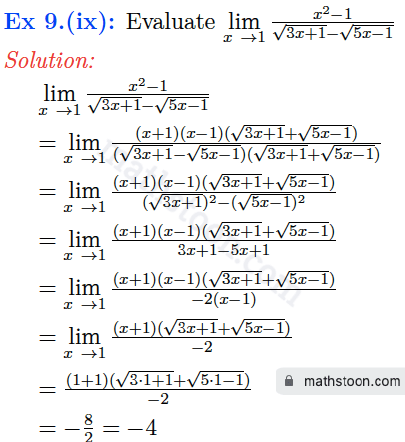SN Dey class 11 Limits very short answer type questions 9.(x) solutions: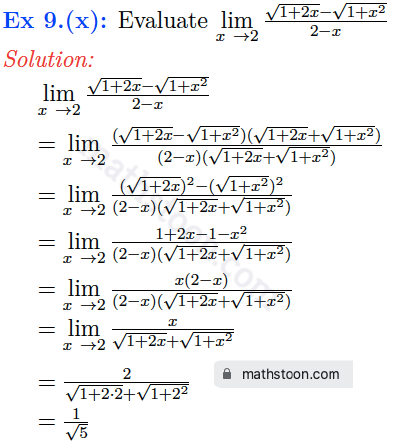SN Dey class 11 Limits very short answer type questions 9.(xi) solutions: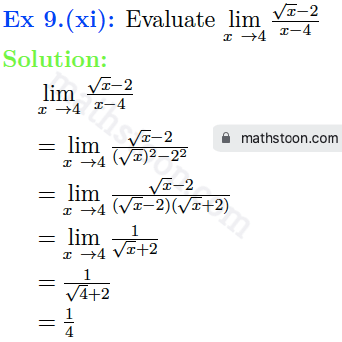SN Dey class 11 Limits very short answer type questions 9.(xii) solutions: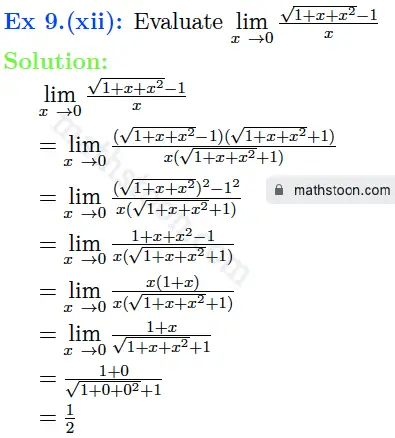SN Dey class 11 Limits very short answer type questions 9.(xiii) solutions: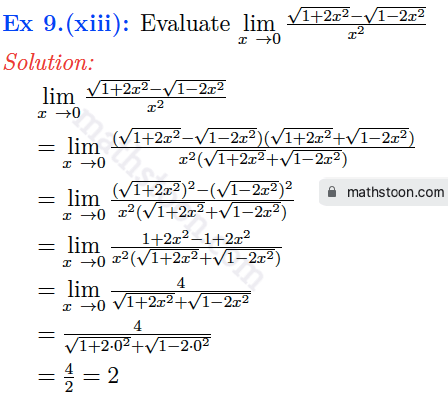SN Dey class 11 Limits very short answer type questions 10.(i) solutions: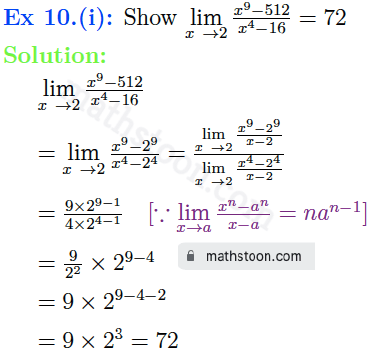SN Dey class 11 Limits very short answer type questions 10.(ii) solutions: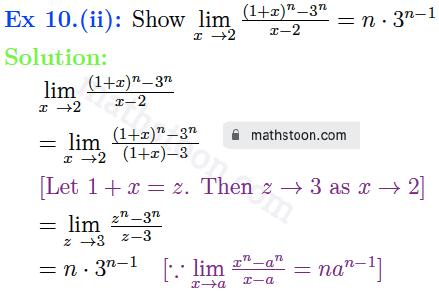SN Dey class 11 Limits very short answer type questions 10.(iii) solutions: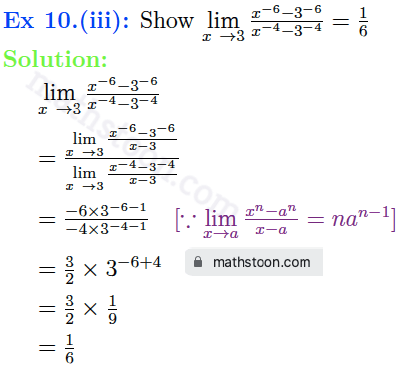SN Dey class 11 Limits very short answer type questions 10.(iv) and (v) solutions: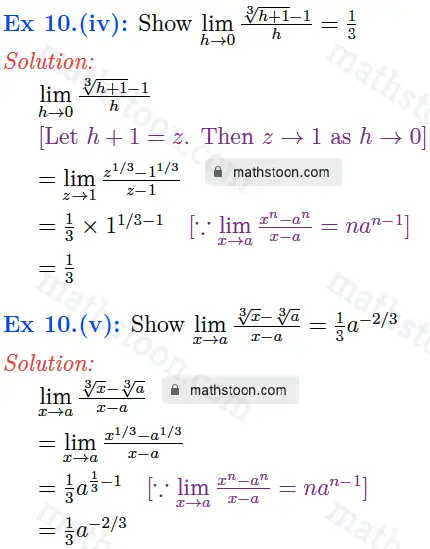SN Dey class 11 Limits very short answer type questions 10.(vi) solutions: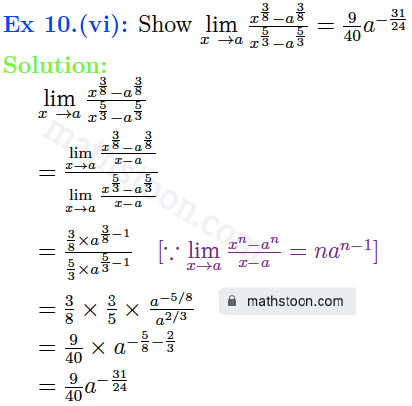SN Dey class 11 Limits very short answer type questions 10.(vii) solutions: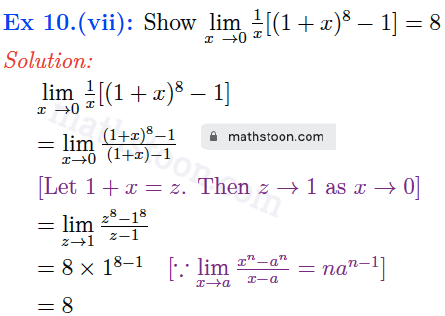SN Dey class 11 Limits very short answer type questions 10.(viii) solutions: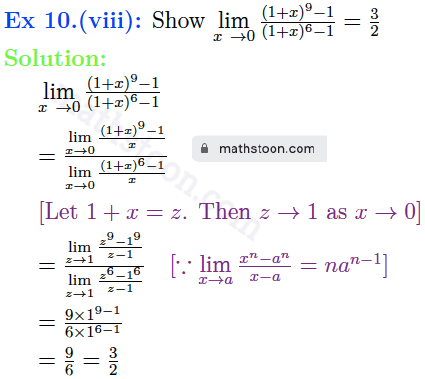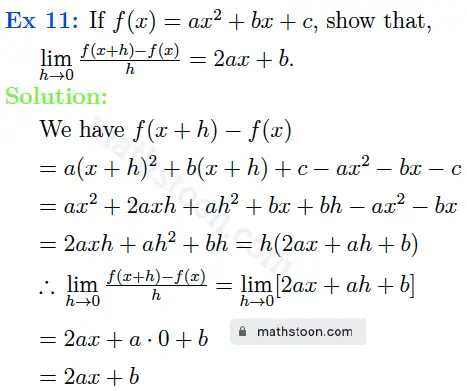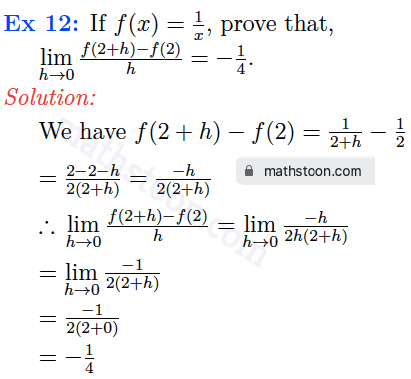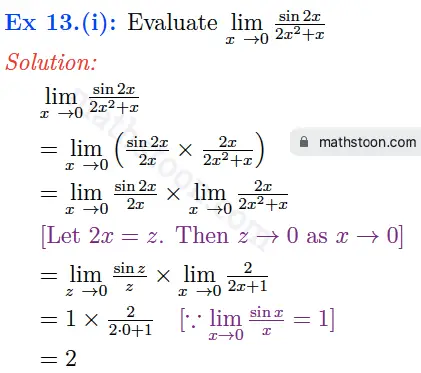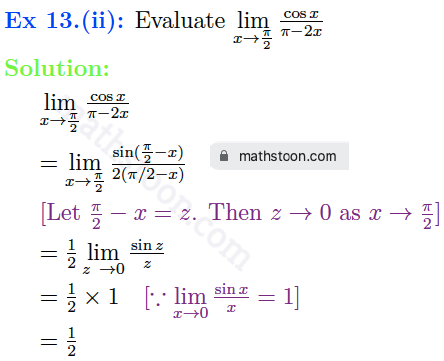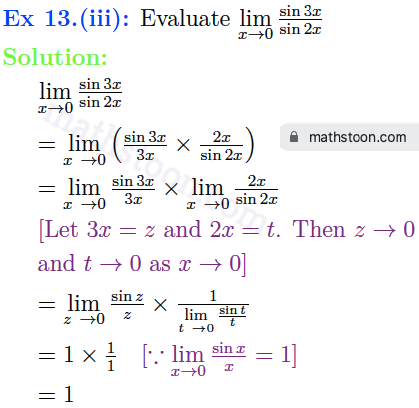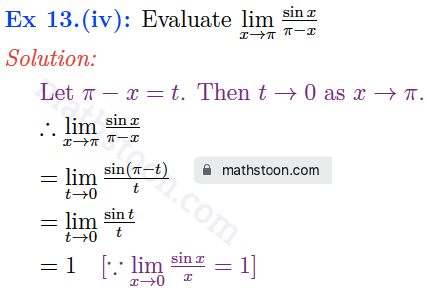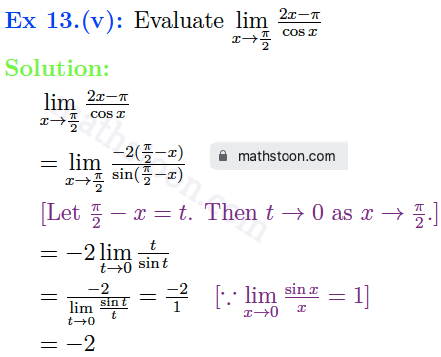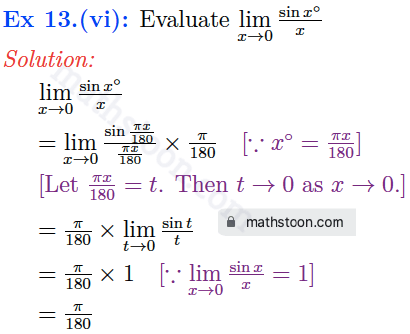Related Topics
Share via: